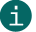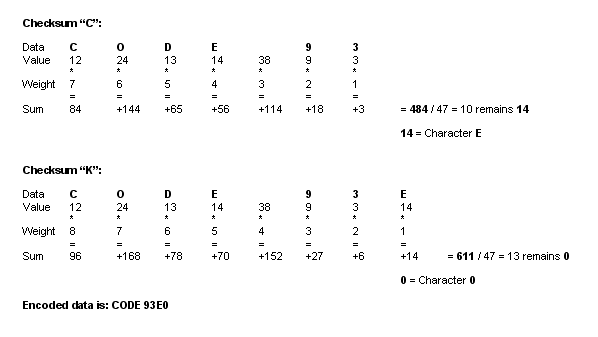# Check digit calculation of Modulo 47

Modulo 47

## This is a description of calculating check digits according to Modulo 47.ActiveBarcode calculates this check digits for most codes automatically.
This documentation is only for the sake of completeness.

Calculation of a checksum according to Modulo 47:
A check character according to Modulo 47 is used e.g. by the Code 93.

Code 93 uses 2 checksums, the so-called "C" checksum and the so-called "K" checksum. Both are calculated according to the same principle. However, the "K" checksum also contains the "C" checksum.

During the calculation, reference numbers are first assigned to all characters of the code. Each digit in the code is assigned a weighting and was incremental from behind. Accordingly, the last position has the weight 1, the penultimate position the weight 2, and so on. The reference numbers are multiplied in each case by the weighting. The resulting individual results are added together.

This total is now divided by 47. The remainder of this division corresponds to the checksum, which is coded as a character using the reference number.

The "K" checksum is determined in the same way. However, this time the character string of the code - as with the first checksum - plus the "C" checksum is used as the basis. The first determined checksum is included in the calculation of the second checksum.

A calculation example:Reference numbers Code 93:

 00 0 01 1 02 2 03 3 04 4 05 5 06 6 07 7 08 8 09 9 10 A 11 B 12 C 13 D 14 E 15 F 16 G 17 H 18 I 19 J 20 K 21 L 22 M 23 N 24 O 25 P 26 Q 27 R 28 S 29 T 30 U 31 V 32 W 33 X 34 Y 35 Z 36 - 37 . 38 Space 39 \$ 40 / 41 + 42 % 43 (\$) 44 (%) 45 (/) 46 (+) - * Start/Stop